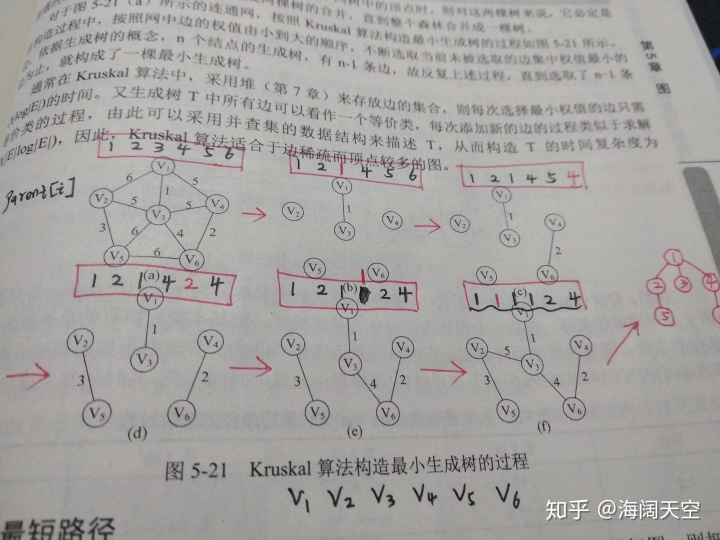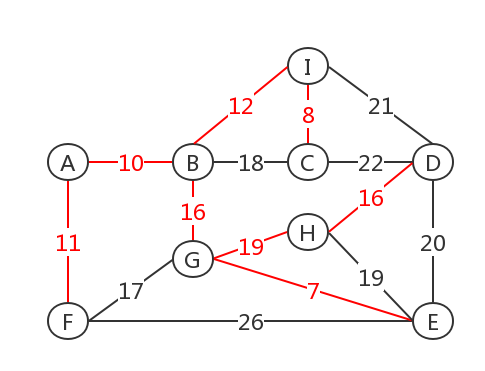• 1.Prim算法 时间是复杂度O（n2），适合稠密图。 例：Poj–1258 题目大意：n个城市建造光缆，要使这些城市直接通信，并且光缆费用最小。 #include #include #define n 10010 #define inf 100010 int a[n][n],ans...
1.Prim算法  时间是复杂度O（n2），适合稠密图。  例：Poj–1258  题目大意：n个城市建造光缆，要使这些城市直接通信，并且光缆费用最小。
#include <cstdio>
#include <string.h>
#define n 10010
#define inf 100010
int a[n][n],ans;
bool vis[n],t;
int dis[n];
bool prim()
{
memset(vis,0,sizeof(vis));
for(int i=1; i<=t; i++)
dis[i]=inf;
ans=0;
dis=0;
for(int i=1; i<=t; i++)
{
int temp=inf,k=0;
for(int j=1; j<=t; j++)
{
if((vis[j]==0)&&(dis[j]<temp))
{
temp =dis[j];
k=j;
}
}
if(temp==n)
return false;
vis[k]=true;
ans+=temp;
for(int j=1; j<=t; j++)
{
if(vis[j]==0&&dis[j]>a[k][j])
dis[j]=a[k][j];
}
}
return true;
}
int main()
{
while(~scanf("%d",&t))
{
ans=0;
for(int i=1; i<=t; i++)
for(int j=1; j<=t; j++)
scanf("%d",&a[i][j]);
prim();
printf("%d\n",ans);
}
return 0;
}
2.Kruskal算法  时间复杂度O（elog2e），适合简单图。  例如：Poj–2485  题目大意：给定一些地点和连通它们的可以修的公路，每条路有不同长度，求修建一些路使它们连通，并且所有路长度最小。
#include <cstdio>
#include <string.h>
#include <algorithm>
using namespace std;
#define N 1001
#define inf 1000000001
#define M N*N
int t;
int e,n,m;
int f[N];
struct note
{
int u,v;
int c;
note() {}
note(int u,int v,int c):u(u),v(v),c(c) {}
} p[M];
{
p[e++]=note (u,v,c);
}
int cmp(const void * a,const void * b)
{
note *aa=(note *)a;
note *bb=(note *)b;
return aa->c-bb->c;
}
{
int i;
for(i=0; i<=n; i++)
f[i]=i;
}
int find(int x)
{
if(x!=f[x])
f[x]=find(f[x]);
return f[x];
}
int  kru(int n)
{
qsort(p,e,sizeof(p),cmp);
int m=0,ans=0;
int i,j;
for(i=0; i<e; i++)
{
int xx=find(p[i].u);
int yy=find(p[i].v);
if(xx==yy)
continue;
m++;
ans =p[i].c;
f[yy]=xx;
if(m==n-1)
break;
}
if(m<n-1)
return -1;
else return ans;
}
int main()
{
int i,j,l,c;;
scanf("%d",&t);
while(t--)
{
scanf("%d",&n);
e=0;
for(i=1; i<=n; i++)
for(j=1; j<=n; j++)
{
scanf("%d",&c);
}
printf("%d\n",kru(n));
}
return 0;
}
展开全文poj
• ## 最小生成树算法

千次阅读 2013-11-24 00:14:24
最小生成树算法是给定一个无向图，及其顶点和边的权值，求最小生成树的算法。主要可以分为prim算法和kruskal算法，prim算法的思想是加点法。即开始时任意从图中选择一个点，作为U集合，其余点为S-U集合，其中S为原图...
最小生成树算法是给定一个无向图，及其顶点和边的权值，在确保所有顶点都是连通即整个无向图的连通分量为1的情况下使得所有边的权值之和最小的算法，这个时候其实图已经退化为树了。
下面介绍一下最小生成树的两种算法：kruskal算法和prim算法。它们都属于贪心算法，只是贪心的理解角度不同导致的两种算法。对于最小生成树，最简单的最优度量准则是：选择迄今为止已入选S中边的代价之和增量最小的边。（其中S代表已经入选最小生成树集合的边）
一）kruskal算法：
贪心准则：按边的权值非降序考察E中的边，从中选择一条代价最小的边。这种做法使得算法在构造生成树的过程中，边集S代表的子图不一定是连通的，而且为了确保最终得到一棵生成树，每选择一条边时都需要判断SUe是否包含回路。时间复杂度为0(eloge)，其中e为图中边的个数，需要结合并查集解决。适合在稀疏图中。
二）prime算法：
贪心准则为：在保证S所代表的子图是一棵树的前提下选择一条最小代价边e=(u,v)。由于其选边方式已经确保S所代表的子图自然是一棵树。故无需判断边集SUe是否包含回路。
时间复杂度为O(n*n)，适合在n比较小的情况下。

两种算法的实现分别如下：
prim算法的思想是加点法。即开始时任意从图中选择一个点，作为U集合，其余点为S-U集合，其中S为原图中的顶点集合，求U集合中顶点到S-U集合中顶点的最小距离，这个最小距离即为最小生成树的组成边，然后将对应最小距离的S-U集合的顶点放入U集合，然后重复执行上述操作，直至U集合=S集合。
而kruskal算法则是加边法，即将图的边先从小到大排序，然后结合并查集依次加边，注意不能形成环，这也是并查集的作用，每次成功加入的边即为最小生成树的组成边。下面是代码：

//图的邻接矩阵表示法
#include <stdio.h>
#include <stdlib.h>
#define Max 100
#define Inf 0x1111
typedef char type;
typedef struct Grap{
type data[Max];
int value[Max][Max];
int n,m;
}Grap,*pgrap;
int index;
int dis;
}trim[Max];
int Located(pgrap g,char ch){
for(int i=0;i<g->n;i++)
if(g->data[i]==ch)
return i;
}
void Creat_grap(pgrap g){
printf("输入图的顶点数和边数:\n");
scanf("%d%d",&g->n,&g->m);
//printf("ksgfdkj\n");
getchar();
printf("输入图中的顶点:\n");
int i,j;
for(i=0;i<g->n;i++){
g->data[i]=getchar();
getchar();
}
for(i=0;i<g->n;i++)
for(j=0;j<g->n;j++)
g->value[i][j]=Inf;
printf("请输入图中的边:\n");
int index1,index2,value;
char ch1,ch2;
while(g->m--){
scanf("%c,%c,%d",&ch1,&ch2,&value);
getchar();
index1=Located(g,ch1);
index2=Located(g,ch2);
g->value[index1][index2]=value;
//无向图
//g->value[index2][index1]=value;
}
}
/*void Show_grap(pgrap g){
printf("邻接矩阵表示法个顶点的邻接顶点:\n");
int i,j;
for(i=0;i<g->n;i++){
printf("%c:",g->data[i]);
for(j=0;j<g->n;j++)
if(g->value[i][j]!=Inf)
putchar(g->data[j]);
printf("\n");
}
}*/
int Minsearch(pgrap g){
int Minn=Inf;
int index;
for(int i=0;i<g->n;i++){
if(trim[i].dis!=0 && trim[i].dis<Minn){
Minn=trim[i].dis;
index=i;
}
}
return index;
}
void MinTreePrim(pgrap g,char ch){
int i,j,index=Located(g,ch);
for(i=0;i<g->n;i++)
if(i!=index){
trim[i].index=index;
trim[i].dis=g->value[index][i];
}
trim[index].dis=0;
int s;
int count=0;
for(i=0;i<g->n-1;i++){
s=Minsearch(g);
count+=trim[s].dis;
printf("%c,%c,%d\n",g->data[s],g->data[trim[s].index],trim[s].dis);
trim[s].dis=0;
for(j=0;j<g->n;j++)
if(trim[j].dis!=0 && g->value[s][j]<trim[j].dis){
trim[j].index=s;
trim[j].dis=g->value[s][j];
}
}
printf("最小生成树的权值为：%d\n",count);
}

int main(){
Grap g;
pgrap p=&g;
Creat_grap(p);
//Show_grap(p);
printf("\n");
MinTreePrim(p,'A');
return 0;
}

测试数据：

输入图的顶点数和边数: 5 7 输入图中的顶点: A B C D E 请输入图中的边: A,B,20 A,C,10 B,D,50 A,D,40 B,E,60 C,D,30 D,E,70   C,A,10 B,A,20 D,C,30 E,B,60 最小生成树的权值为：120     Terminated with return code 0 Press any key to continue ...
下面是kruskal算法代码：

//最小生成树kruskal算法
#include <stdio.h>
#include <stdlib.h>
#include <algorithm>
#define Max(a,b) a>b?(a):(b)
#define Min(a,b) a<b?(a):(b)
#define Maxnode 100 //顶点的最大个数
#define Maxarc 200 //边的最大个数
struct Arcnode{
int from;
int to;
int value;
}arcnode[Maxarc];
int set[Maxnode];
int n,m;
int cmp(const void *p,const void *q){
return ((Arcnode*)p)->value-((Arcnode*)q)->value;
}
int find(int x){
int a=x,j;
while(set[x]!=x)
x=set[x];
j=a;
while(j!=x){
a=set[j];
set[j]=x;
j=a;
}
return x;
}
int main(){
printf("请输入图的顶点数和边数:\n");
scanf("%d%d",&n,&m);
int i,x,y;
for(i=0;i<n;i++)
set[i]=i;
printf("请输入各边及其权值:\n");
for(i=0;i<m;i++)
scanf("%d%d%d",&arcnode[i].from,&arcnode[i].to,&arcnode[i].value);
qsort(arcnode,m,sizeof(Arcnode),cmp);
int count=0;
for(i=0;i<m;i++){
x=find(arcnode[i].from);
y=find(arcnode[i].to);
if(x!=y){
set[Max(x,y)]=Min(x,y);
count+=arcnode[i].value;
}
}
printf("最小生成树权值为:%d\n",count);
return 0;
}

测试数据：

请输入图的顶点数和边数: 4 5 请输入各边及其权值: 0 2 20 0 1 10 1 3 30 2 3 50 0 3 40 最小生成树权值为:60     Terminated with return code 0 Press any key to continue ...  下面以poj1258为例分别用kruskal算法和prime算法
kruskal算法如下：288K+16MS
#include <stdio.h>
#include <stdlib.h>
#include <string.h>
#include <algorithm>
#define Maxx(a,b) (a>b)?(a):(b)
#define Min(a,b) (a<b)?(a):(b)
#define Max 110
using namespace std;
int set[Max];
struct Node{
int from,to;
int value;
}node[Max*Max];
int n;
int find(int x){
int j=x;
while(set[x]!=x)
x=set[x];
int temp;
while(j!=x){
temp=set[j];
set[j]=x;
j=temp;
}
return x;
}
bool cmp(const struct Node p,const struct Node q){
return p.value<q.value;
}
int main(){
while(scanf("%d",&n)!=EOF){
int pivot=0;
for(int i=1;i<=n;i++){
set[i]=i;
for(int j=1;j<=n;j++){
node[pivot].from=i;
node[pivot].to=j;
scanf("%d",&node[pivot++].value);
}
}
sort(node,node+pivot,cmp);
int Sum=0;
for(int i=0;i<pivot;i++){
int x=find(node[i].from);
int y=find(node[i].to);
if(x!=y){
set[Maxx(x,y)]=Min(x,y);
Sum+=node[i].value;
}
}
printf("%d\n",Sum);
}
return 0;
}

下面是prim算法：212K+0MS
#include <stdio.h>
#include <stdlib.h>
#include <string.h>
#define Max 110
#define Inf 10000010
int dis[Max];
int trim[Max][Max];
bool flag[Max];
int n;
int find_min(){
int max_int=Inf,index;
for(int i=1;i<=n;i++)
if(!flag[i] && dis[i]<max_int){
max_int=dis[i];
index=i;
}
return index;
}
void prim(){
memset(flag,0,sizeof(flag));
for(int i=1;i<=n;i++)
dis[i]=trim[i];
flag=true;
int Sum=0;
for(int i=1;i<n;i++){
int index=find_min();
Sum+=dis[index];
flag[index]=true;
for(int j=1;j<=n;j++)
if(!flag[j] && trim[index][j]<dis[j])
dis[j]=trim[index][j];
}
printf("%d\n",Sum);
}
int main(){
while(scanf("%d",&n)!=EOF){
for(int i=1;i<=n;i++)
for(int j=1;j<=n;j++)
scanf("%d",&trim[i][j]);
prim();
}
return 0;
}


展开全文• Java: Kruskal算法生成最小生成树(邻接矩阵):package 输出：Kruskal=36: (E,F) (C,D) (D,E) (B,F) (E,G) (A,B) 分析：Java: Kruskal算法生成最小生成树(邻接矩阵) 克鲁斯卡尔(Kruskal)算法 Kruskal算法和Prim算法...

Java: Kruskal算法生成最小生成树(邻接矩阵):

package 

输出：
Kruskal=36: (E,F) (C,D) (D,E) (B,F) (E,G) (A,B)
分析：

Java: Kruskal算法生成最小生成树(邻接矩阵)
克鲁斯卡尔(Kruskal)算法
Kruskal算法和Prim算法相比，就是Kruskal算法从边出发，不断寻找当前未添加进Et的、且权值最小的边，若添加后不形成环，则添加成功；
因为形成环，说明已经是连同了，这条边是不需要的。否则跳过，
继续尝试添加下一条边。最后，判断边的数量arcnum是否是点的数量vexnum-1，若是则最小生成树构造成功，否则失败。
Prim算法与顶点相关时间复杂度O（|V|2），所以适合顶点少边多的图；
Kruskal反之，算法与边相关，时间复杂度为O（|E|log|E|），所以适合边少顶点多的图；


图解：展开全文• Prim算法：假设N=(V,E) 是具有n个顶点的连通图，设U是最小生成树中顶点的集合，设TE是最小生成树中边的集合；  初始，U = { u1 } ，TE = { } ，  重复执行: 在所有 u∈U，v∈V-U 的边 ( u , v...
最小生成树（MST）：对于带权无向图所有的生成树中，代价最小的生成树称为图的最小生成树。
Prim算法：假设N=(V,E) 是具有n个顶点的连通图，设U是最小生成树中顶点的集合，设TE是最小生成树中边的集合；
初始，U = { u1 } ，TE = { } ，
重复执行: 在所有 u∈U，v∈V-U 的边 ( u , v ) 中寻找代价最小的边( u’ , v’ ) ，并纳入集合 TE 中；
同时将 v’ 纳入集合 U 中；
直至 U = V 为止。
Prim算法也是典型的贪心算法。最小生成树的主要有两个重复过程：寻找一个满足条件的未插入到生成树集合的结点；利用该结点更新其余顶点到生成树集合的最小权值信息。因此，时间复杂度为O()，其中n为图的结点。
如下图prim算法过程：初始U={A},V-U={B,C,D,E,F,G,H,I}     TE={}
U={A,B},V-U={C,D,E,F,G,H,I}      +(A,B)
U={A,B,F},V-U={C,D,E,G,H,I}      +(A,F)
U={A.B,F,I},V-U={C,D,E,G,H}      +(B,I)
U={A,B,F,I,C},V-U={D,E,G,H}      +(I,C)
U={A,B,F,I,C,G},V-U={D,E,H}      +(B,G)
U={A,B,F,I,C,G,E},V-U={D,H}      +(G,E)
U={A,B,F,I,C,G,E,H},V-U={D}      +(G,H)
U={A,B,F,I,C,G,E,H,D},V-U={}     +(H,D)
代码实现

import sys
if __name__=='__main__':
MAX = sys.maxsize
primgraph = [[MAX,  10, MAX, MAX, MAX,  11, MAX, MAX, MAX],
[10,  MAX,  18, MAX, MAX, MAX,  16, MAX,  12],
[MAX,  18, MAX,  22, MAX, MAX, MAX, MAX,   8],
[MAX, MAX,  22, MAX,  20, MAX, MAX,  16,  21],
[MAX, MAX, MAX,  20, MAX,  26,   7,  19, MAX],
[11,  MAX, MAX, MAX,  26, MAX,  17, MAX, MAX],
[MAX,  16, MAX, MAX,   7,  17, MAX,  19, MAX],
[MAX, MAX, MAX,  16,  19, MAX,  19, MAX, MAX],
[MAX,  12,   8,  21, MAX, MAX, MAX, MAX, MAX]]
chararray = ['A','B','C','D','E','F','G','H','I']
charlist = []
charlist.append(chararray)
mid = []    #mid[i]表示生成树集合中与点i最近的点的编号
lowcost = []    #lowcost[i]表示生成树集合中与点i最近的点构成的边最小权值 ，-1表示i已经在生成树集合中
lowcost.append(-1)
mid.append(0)
n = len(chararray)
for i in range(1,n): #初始化mid数组和lowcost数组
lowcost.append(primgraph[i])
mid.append(0)
sum = 0
for _ in range(1,n): #插入n-1个结点
minid = 0
min = MAX
for j in range(1,n):  #寻找每次插入生成树的权值最小的结点
if(lowcost[j]!=-1 and lowcost[j]<min):
minid = j
min = lowcost[j]
charlist.append(chararray[minid])
print(chararray[mid[minid]]+'——'+chararray[minid]+'权值：'+str(lowcost[minid]))
sum+=min
lowcost[minid] = -1
for j in range(1,n):  #更新插入结点后lowcost数组和mid数组值
if(lowcost[j]!=-1 and lowcost[j]>primgraph[minid][j]):
lowcost[j] = primgraph[minid][j]
mid[j] = minid
print("sum="+str(sum))
print("插入结点顺序："+str(charlist))
运行结果
A——B权值：10
A——F权值：11
B——I权值：12
I——C权值：8
B——G权值：16
G——E权值：7
G——H权值：19
H——D权值：16
sum=99
插入结点顺序：['A', 'B', 'F', 'I', 'C', 'G', 'E', 'H', 'D']


展开全文Python
• 最小生成树算法Prim & Kruskal ，时间复杂度 O（VlgE）
• Prime算法  分类： 算法总结2011-...普利姆算法最小生成树时候，和边数无关，只和定点的数量相关，所以适合求稠密网的最小生成树，时间复杂度为O（n*n）。 算法过程： 1.将一个图的顶点分为两部分，一部
• 最小生成树算法总结 Kruskal算法 Kruskal算法是典型的最小生成树算法，用于计算将所有顶点连通的最小权值。 最常见的问题就是：已知N座城市中任意两座城市之间建造道路所需要的费用，求最少花费多少就可以使得...
• 最小生成树的应用场景很广，例如电信公司需要将9个村庄进行网络连接，村庄间的距离都不相同，怎么连接才能达到成本最小了？村庄结构图如下： V0-V10分别表示村庄，节点间的权重代表距离，连接所有节点的总距离最小...Prim Kruskal Python
• ## prim最小生成树算法原理

万次阅读 多人点赞 2016-05-03 16:51:26
prim 最小生成树算法原理 主要需要了解算法的原理、算法复杂度、优缺点 、刻画和度量指标 评价等 可以查阅相关的文献，这部分内容主要整合了两篇博客的内容 分别是：...
• c++描述的数据结构算法中的prim最小生成树算法，利用最小堆来实现时间复杂度为O(elog2e)大家多多支持哦！！！
• 最小生成树算法之KrusKal 在上一章中，我们已经介绍了最小生成树的算法之一Prim算法，该算法是一个贪心算法，主要是随机选一个开始点，在找出与这个点相关的最小边，然后加入到生成树中，然后在一次重复上述过程，...kruskal prim
• 首先，我们要知道，图的最小生成树是针对于有权图而言的，笔者的上一篇文章只介绍了无权图，其实有权图和无权图唯一的区别就是有权图的边是有权值的，不同的边权值可以不同，对于无权图我们可以把它看成所有边的权值...
• 遗传算法与最小生成 树算法解决旅行商问 题分析对比 摘要 本实验采用遗传算法实现了旅行商问题的模拟求解并在同等规 模问题上用最小生成树算法做了一定的对比工作遗传算法在计算时 间和占用内存上都远远优于最小生成...
• 生成树：连通图中取出一个...求最小生成树算法主要有两个： prim算法和kruskal算法，那么我们接下来分别来了解一下： --------------------------------------------------prim算法----------------------------C++
• 普里姆算法(Prim’s algorithm)是求出最小生成树的算法, 也就是在包含 n个顶点的带权无向连通图中, 找出(n-1)条边的最小耗费生成树(Minimum Cost Spanning Tree), 简称 MST 普里姆算法的时间复杂度为: 邻接矩阵 O(v^...普里姆算法 Prim MST
• Kruskal算法：  算法过程：  1、将各条边按照权值从大到小排列 ...递归重复步骤2，直到找出n-1条边为止（设图有n个结点，则最小生成树的边数应为n-1条），算法结束。得到的就是此图的最小生成树kruskal prim
• 什么是生成树？ 一个连通图的生成树是一个极小的连通子图，它含有图中全部的n个顶点，但是只有足以构成一棵树的n-1条边。 理解： 连通图是属于无向图的范畴，有向图的连通子图叫强连通图 它含有n个全部顶点，...无向网
• 最小生成树 最小生成树（minimum spanning tree）是由n个顶点，n-1条边，将一个连通图连接起来，且使权值最小的结构。 最小生成树可以用Prim（普里姆）算法或kruskal（克鲁斯卡尔）算法求出。 我们将以下面的带权...
• Kruskal算法与Prime算法的区别就在于一个是以边为目标进行考虑，一个以点为目标进行考虑。 由于Kruskal算法以边进行考虑，就涉及到边可能属于两个连通块，这时候就涉及到连通块的判断查找合并。 这个知识点属于 ...
• 主要就是以点作为目标来考虑， 而不是以边进行考虑。 由于思想一样，所以时间复杂度分析的结果与Dijkstra算法的一模一样。
• 较为完全的初学者学习最小生成树的利器，想要玩转ACM的小编倾情奉献...